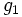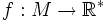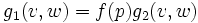Conformally equivalent metrics

Let$g_1$ and$g_2$ be two Riemannian metrics on a differential manifold$M$. Then we say that$g_1$ is conformally equivalent to$g_2$ if there is a scalar function$f:M \to \mathbb{R}^*$ such that for any$p \in M$, and tangent vectors$v,w \in T_pM$:$g_1(v,w) = f(p)g_2(v,w)$